Ex 15.1

Chapter 15 Class 10 Probability
Serial order wise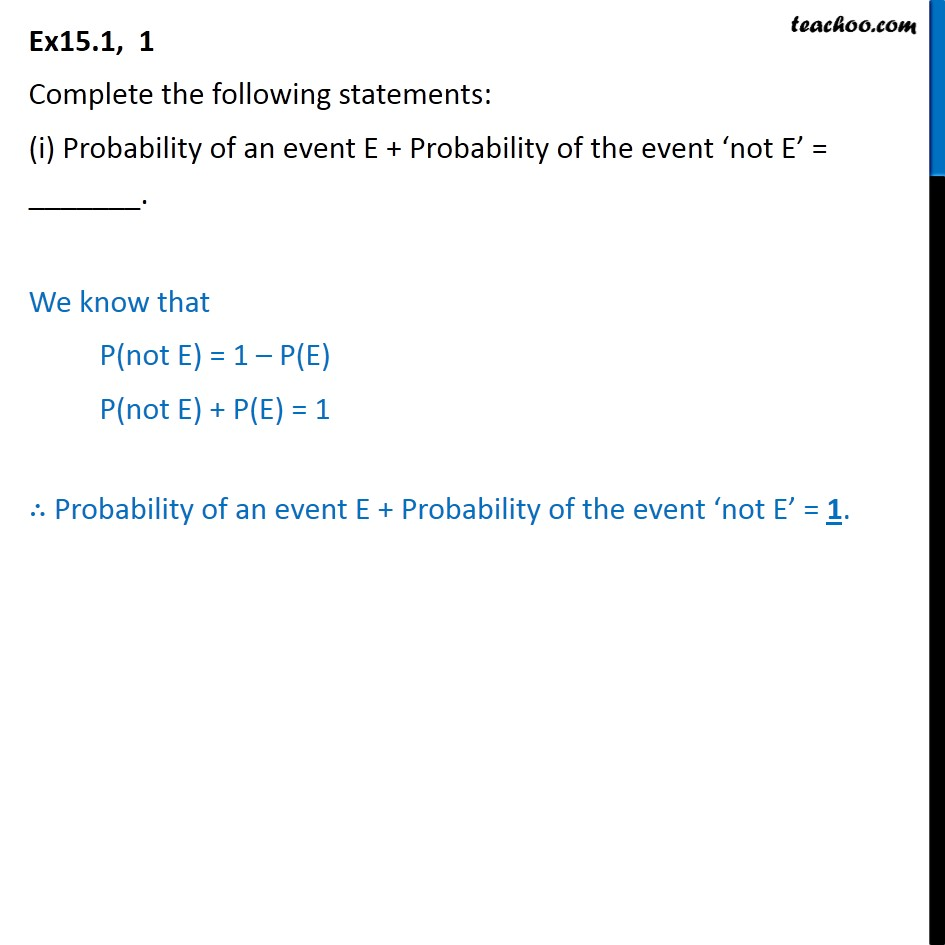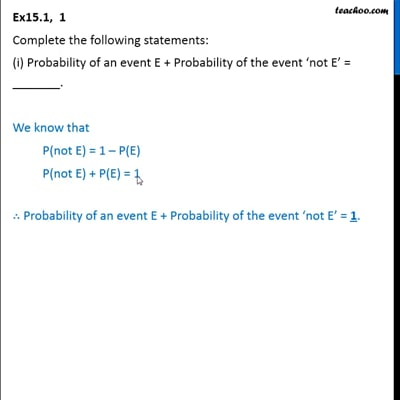This video is only available for Teachoo black users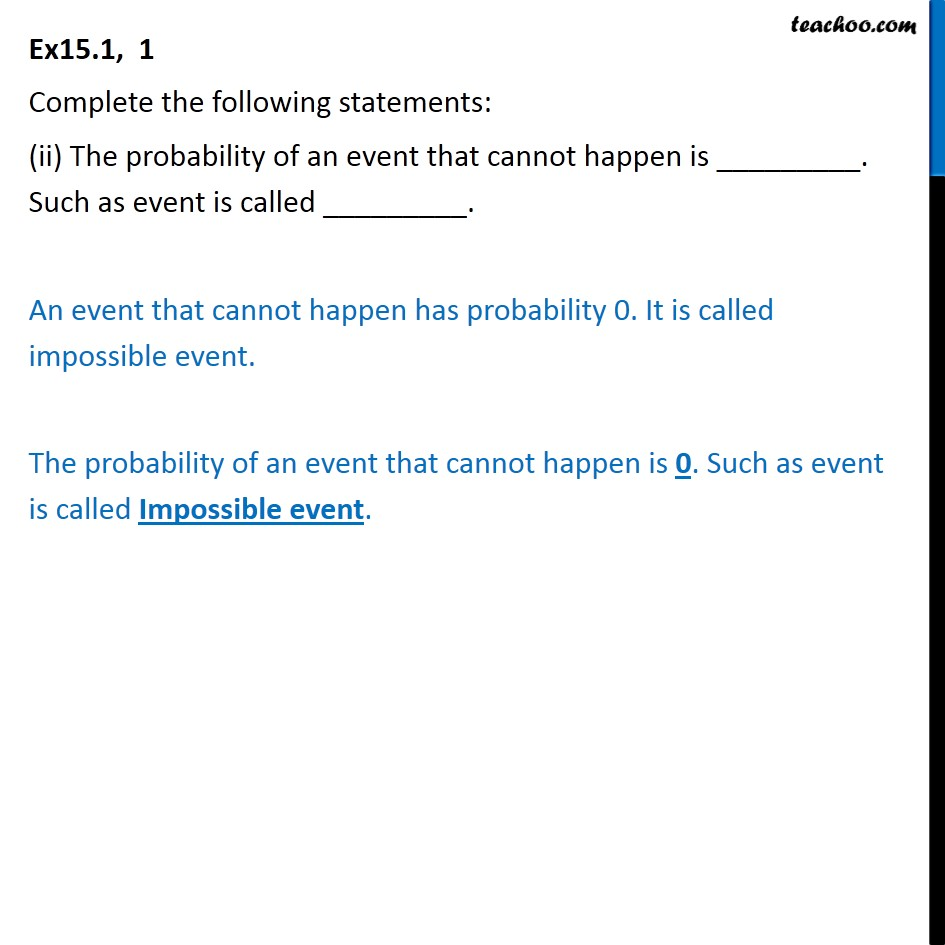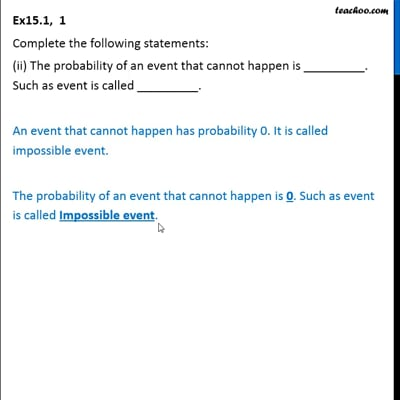This video is only available for Teachoo black users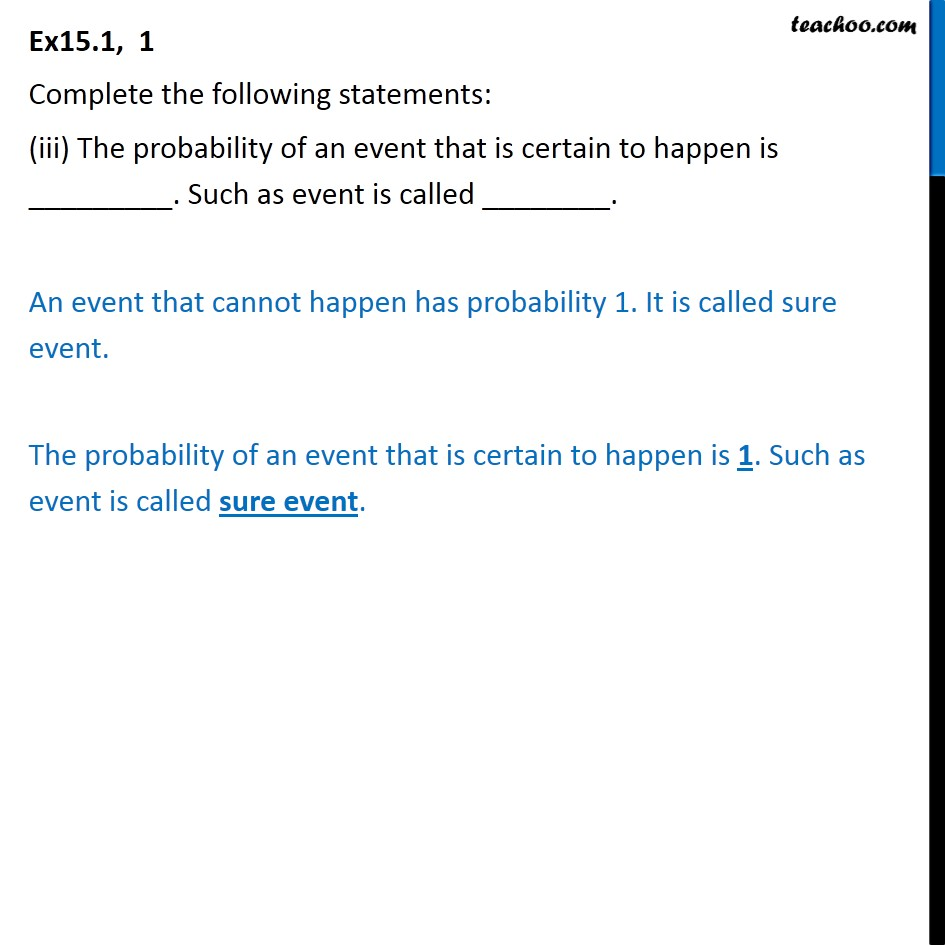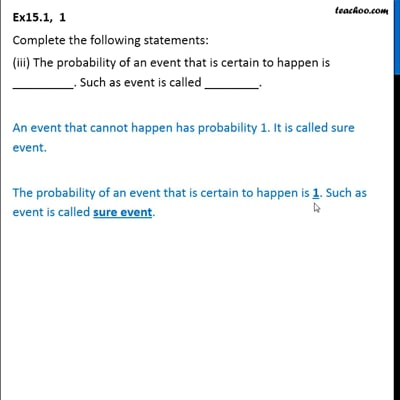This video is only available for Teachoo black users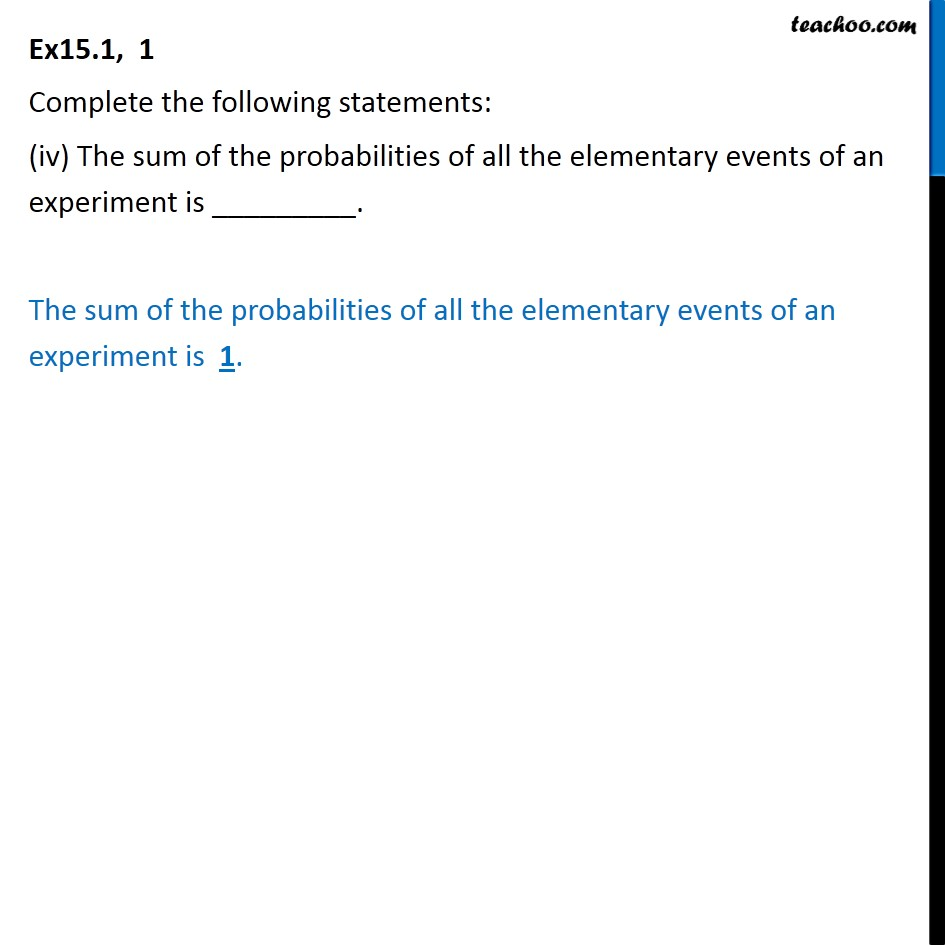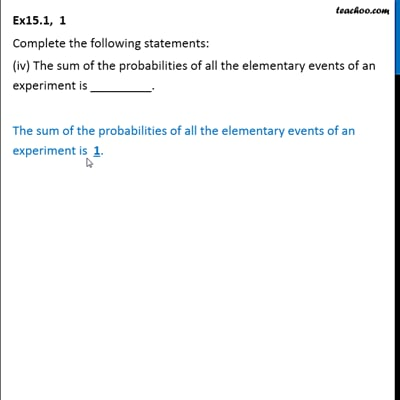This video is only available for Teachoo black users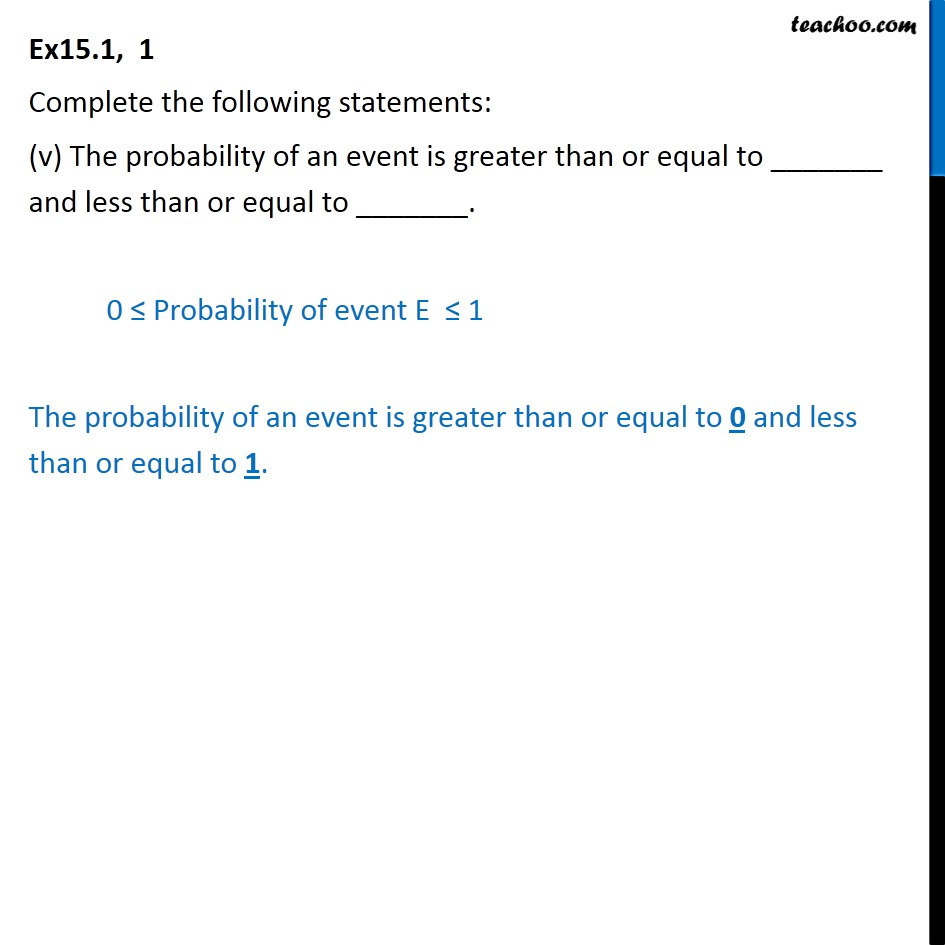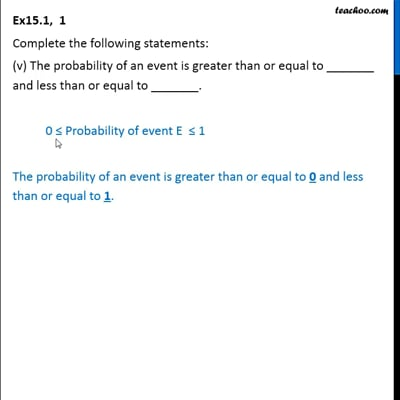This video is only available for Teachoo black users

Solve all your doubts with Teachoo Black (new monthly pack available now!)

### Transcript

Ex15.1, 1 Complete the following statements: (i) Probability of an event E + Probability of the event not E = _______. We know that P(not E) = 1 P(E) P(not E) + P(E) = 1 Probability of an event E + Probability of the event not E = 1. Ex15.1, 1 Complete the following statements: (ii) The probability of an event that cannot happen is _________. Such as event is called _________. An event that cannot happen has probability 0. It is called impossible event. The probability of an event that cannot happen is 0. Such as event is called Impossible event. Ex15.1, 1 Complete the following statements: (iii) The probability of an event that is certain to happen is _________. Such as event is called ________. An event that cannot happen has probability 1. It is called sure event. The probability of an event that is certain to happen is 1. Such as event is called sure event. Ex15.1, 1 Complete the following statements: (iv) The sum of the probabilities of all the elementary events of an experiment is _________. The sum of the probabilities of all the elementary events of an experiment is 1. Ex15.1, 1 Complete the following statements: (v) The probability of an event is greater than or equal to _______ and less than or equal to _______. 0 Probability of event E 1 The probability of an event is greater than or equal to 0 and less than or equal to 1.